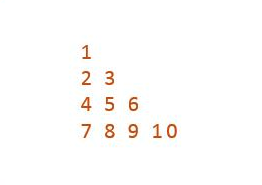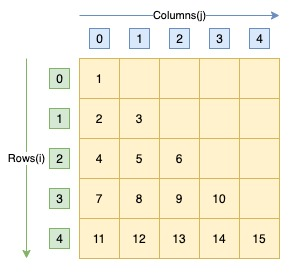# C Program to Print Natural Numbers in Right Triangle Pattern

Here is a C program to print natural numbers right angled triangle. For a right triangle of 4 rows. Program's output should be:#### Required Knowledge

Algorithm to print natural number right triangle pattern using for loop
• Take the number of rows(N) of right triangle as input from user using scanf function.
• Number of integers in Kth row is always K. 1st row contains 1 integer, 2nd row contains 2 integers, 3rd row contains 3 integers and so on. In general, Kth row contains K integers.
• We will use a integer counter to print consecutive natural numbers.
• We will use two for loops to print right triangle of natural numbers.
• Outer for loop will iterate N time. Each iteration of outer loop will print one row of the pattern.
• For Kth row of right triangle pattern, inner loop will iterate K times. Each iteration of inner loop will increment the value of counter and print it on screen.

Here is the matrix representation of the natural number triangle pattern. The row numbers are represented by i whereas column numbers are represented by j.## C program to print right triangle star pattern

```#include<stdio.h>

int main() {
int i, j, rows, count = 1;

printf("Enter the number of rows\n");
scanf("%d", &rows);

for (i = 0; i < rows; i++) {
for (j = 0; j <= i; j++) {
printf("%d ", count);
count++;
}
printf("\n");
}
return(0);
}
```
Output
```Enter the number of rows
4
1
2 3
4 5 6
7 8 9 10
```

Related Topics
 C program binary triangle pattern C program prime number triangle pattern C program palindrome triangle pattern C program right triangle star pattern C program inverted right triangle pattern C program multiplication table triangle pattern C program heart shape star pattern C program pyramid star pattern C program rhombus star pattern List of all C pattern programs